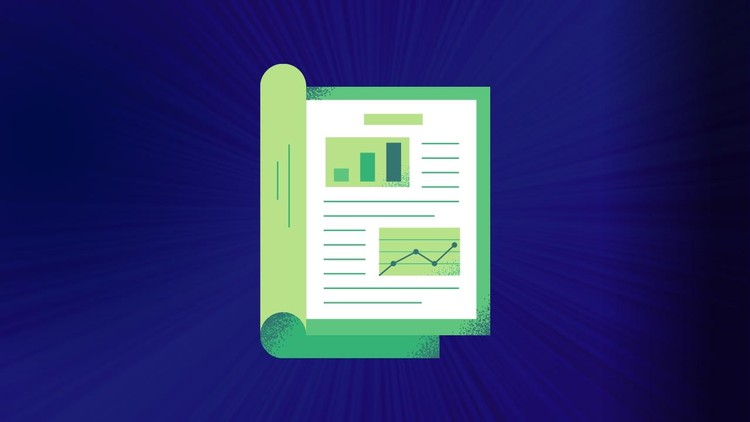All Courses

# Assets, liabilities and the accounting equation## Assets, liabilities and the accounting equation

### Start Learning Accounting

#### What you’ll learn

Assets, liabilities and the accounting equation

• Assets and liabilities
• The accounting equation
• Accounts payable and accounts receivable
• Double entry bookkeeping
• Capital expenditure and revenue expenditure

#### Requirements

• there are no basic requirements, this course is for everyone.

#### Description

You must acquire a thorough understanding of the principles of double-entry bookkeeping. These principles, outlined in this course, apply to both cash and credit transactions.

The purpose, of the Course, is to introduce the fundamentals of accounting, particularly the principles of double-entry bookkeeping. You must understand the topics discussed in the next few chapters, as they form a basis for your studies of financial accounting

#### We will discuss the following topics:

• Assets and liabilities
• The accounting equation
• Accounts payable and accounts receivable
• Double entry bookkeeping
• Capital expenditure and revenue expenditure

The accounting equation is a basic principle of accounting and a fundamental element of the balance sheet. The equation is as follows:

#### Assets = Liabilities + Shareholder’s Equity

This equation sets the foundation of double-entry accounting, also known as double-entry bookkeeping, and highlights the structure of the balance sheet. Double-entry accounting is a system where every transaction affects at least two accounts.

Journal entries often use the language of debits (DR) and credits (CR). Debit refers to an increase in an asset or a decrease in a liability or shareholders’ equity. A credit in contrast refers to a decrease in an asset or an increase in a liability or shareholders’ equity.

The accounting equation forms the basis for the balance sheet. The balance sheet is also sometimes referred to as the statement of financial position. The balance sheet is broken down into three major sections and their various underlying items: Assets, Liabilities, and Shareholder’s Equity.

#### Who this course is for:

• Beginner accounting students and accountants

#### Assets, liabilities and the accounting equation

Bookkeeping and Accounting – Free Udemy Courses